### Simple interface material - obsolete

This model provides a simple interface law with penalty-type contact and friction. In the normal direction, the response is linear elastic, but with different stiffnesses in tension and in compression (stiffness kn in compression, kn*stiffcoeff in tension). By setting kn to a high value, the penetration (overlap) can be reduced, in the sense of the penalty approach. By setting stiffcoeff to 0, free opening of the gap can be allowed. The shear response is elastoplastic, with the yield limit dependent on the normal traction. Crosssection's width, height or area have no influence on the results. The magnitude of the shear traction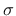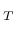must not exceed the yield limit computed according to a Coulomb-like friction law as the product of the (negative part of) normal traction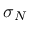and a dimensionless coefficient of friction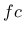: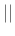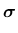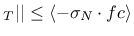(230)is computed multiplying a known normal strain and a known stiffness in tension or in compression.

The shear elastic stiffness is assumed to be kn (i.e., equal to the normal stiffness). If the normal traction is tensile or zero, no shear traction can be transmitted by the interface. In the future, this law will be enriched by a cohesion-like term.

If used in connection of two nodes with interface1delement, the element-level parameter normal determines what is compression and what is tension (from the 1st node to the 2nd node in normal direction it is tension). If normalClearance is defined, the element has tensile stiffness until the gap within the element is closed. This feature allows simulating two faces with a gap. When the gap is smaller than normalClearance, the element stiffness changes to compression, see Figure 11.

The model description and parameters are summarized in Tab. 49.

Table 49: Simple interface material - summary.
 Description Simple interface material Record Format simpleintermat (in) # kn(rn) # [ fc(rn) #] [ stiffcoeff(rn) #] [ normalClearance(rn) #] Parameters - material number - kn (penalty) stiffness in compression - fc friction coefficient - stiffcoeff ratio (tensile stiffness / compression stiffness) - normalClearance free distance within element Supported modes _1dInterfaceBorek Patzak
2019-03-19